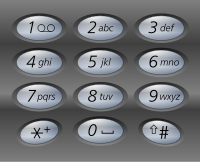# Description

Given a digit string, return all possible letter combinations that the number could represent.

A mapping of digit to letters (just like on the telephone buttons) is given below.Input:Digit string “23”
Output: [“ad”, “ae”, “af”, “bd”, “be”, “bf”, “cd”, “ce”, “cf”].

The original problem is here.

The original code is here.

# My Solution

I solve this problem in C++, as below:

``````/*
*Letter Combinations of a Phone Number
*Author: shuaijiang
*Email: zhaoshuaijiang8@gmail.com
*/
#include<iostream>
#include<vector>
#include<stdlib.h>
using namespace std;

class Solution {
public:
vector<string> letterCombinations(string digits) {
vector<string> res;
string letter[] = {"","","abc","def","ghi","jkl","mno","pqrs","tuv","wxyz"};
int size = digits.size();
if(size <= 0)
return res;
string firstStr = letter[digits-'0'];
for(int i=0;i<firstStr.size();i++){
string oneStr;
oneStr.push_back(firstStr[i]);
res.push_back(oneStr);
}

for(int i=1;i<size;i++){
if(digits[i] == '0'  || digits[i] == '1')
continue;
else {
vector<string> vec;
for(int j=0; j<res.size();j++){
string str = letter[digits[i]-'0'];
for(int k=0;k<str.size();k++){
string oneRes = res[j];
oneRes.push_back(str[k]);
vec.push_back(oneRes);
}
}
res = vec;
}
}
return res;
}
};
``````

# Note

To solve the problem, we just traversal the digits, and add every possible letter to the combinations.